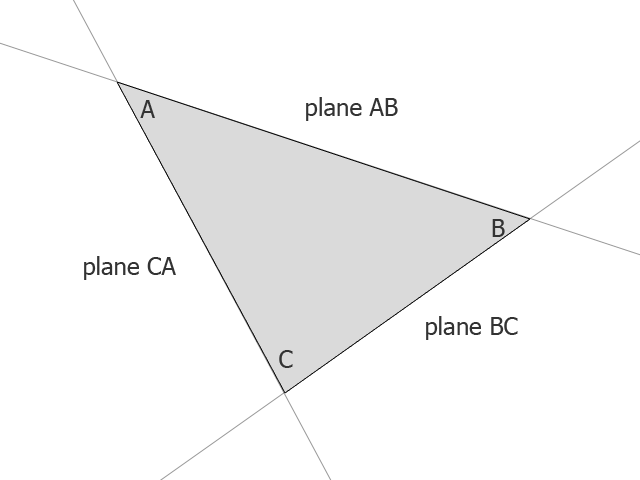# Algorithm to determine if a point is inside a triangle with mathematics (no hit test involved)

A couple of months ago I published an algorithm to determine if a point is inside a square with mathematics (no hit test involved).

Are you ready for some more maths? This time I am publishing an algorithm to determine if a point is inside a triangle.

Although checking if a point is inside “something” may seem just a programming exercise, during next days I will show you how many awesome things you can do with it.

The idea is simple. Given an ABC triangle, every side cuts the plane in two. We can say a point is inside a triangle when its position in all three planes is on the same side of the triangle, or when it’s not in any plane on the opposite side of the triangle.

Look at this picture:We can say a point is inside the triangle when it’s outside of plane AB, outside plane BC and outside plane CA, or inside “rest of the world”-plane AB, inside “rest of the world”-plane BC and inside “rest of the world”-plane CA.

This is what we are going to do:

```package {
import flash.display.Sprite;
import flash.geom.Point;
import flash.events.Event;
import flash.events.MouseEvent;
public class Main extends Sprite {
private var A:Point;
private var B:Point;
private var C:Point;
var triangleCanvas:Sprite=new Sprite();
public function Main() {
triangleCanvas=new Sprite();
drawTriangle(null);
}
private function drawTriangle(e:MouseEvent):void {
A=new Point(Math.random()*600+20,Math.random()*440+20);
B=new Point(Math.random()*600+20,Math.random()*440+20);
C=new Point(Math.random()*600+20,Math.random()*440+20);
}
private function update(e:Event):void {
var mousePoint:Point=new Point(mouseX,mouseY);
triangleCanvas.graphics.clear();
if (isInsideTriangle(A,B,C,mousePoint)) {
triangleCanvas.graphics.lineStyle(1,0xFF0000);
}
else {
triangleCanvas.graphics.lineStyle(1,0x000000);
}
triangleCanvas.graphics.moveTo(A.x,A.y);
triangleCanvas.graphics.lineTo(B.x,B.y);
triangleCanvas.graphics.lineTo(C.x,C.y);
triangleCanvas.graphics.lineTo(A.x,A.y);
}
private function isInsideTriangle(A:Point,B:Point,C:Point,P:Point):Boolean {
var planeAB:Number = (A.x-P.x)*(B.y-P.y)-(B.x-P.x)*(A.y-P.y);
var planeBC:Number = (B.x-P.x)*(C.y-P.y)-(C.x - P.x)*(B.y-P.y);
var planeCA:Number = (C.x-P.x)*(A.y-P.y)-(A.x - P.x)*(C.y-P.y);
return sign(planeAB)==sign(planeBC) && sign(planeBC)==sign(planeCA);
}
private function sign(n:Number):int {
return Math.abs(n)/n;
}
}
}```

And this is the result: click the mouse to draw a random triangle, move the mouse inside the triangle to turn it red.

214 GAME PROTOTYPES EXPLAINED WITH SOURCE CODE
// 1+2=3
// 10000000
// 2 Cars
// 2048
// Avoider
// Ballz
// Block it
// Blockage
// Bloons
// Boids
// Bombuzal
// Breakout
// Bricks
// Columns
// CubesOut
// Dots
// DROP'd
// Dudeski
// Eskiv
// Filler
// Fling
// Globe
// HookPod
// Hundreds
// InkTd
// Iromeku
// Lumines
// Magick
// MagOrMin
// Maze
// Memdot
// Nano War
// Nodes
// o:anquan
// Ononmin
// Pacco
// Phyballs
// Platform
// Poker
// Pool
// Poux
// Pudi
// qomp
// Racing
// Renju
// SameGame
// Security
// Sling
// Slingy
// Sokoban
// Splitter
// Sproing
// Stack
// Stringy
// Sudoku
// Tetris
// Threes
// Toony
// Turn
// TwinSpin
// vvvvvv
// Wordle
// Worms
// Yanga
// Zhed
// zNumbers